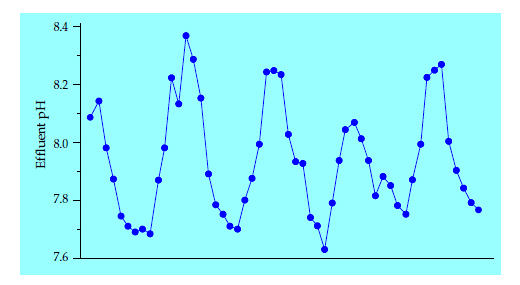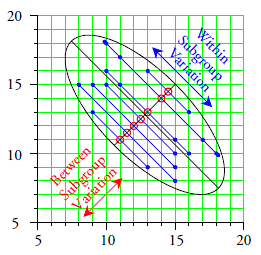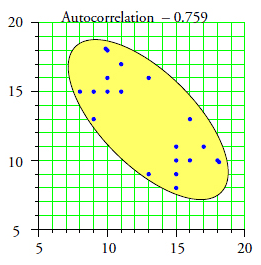Quality Digest

Featured Product
This Week in Quality Digest Live
Statistics Features
Anthony D. Burns
Most people are confused about what quality means. Hopefully, better education in the coming decades will correct this.
Donald J. Wheeler
One of the fundamental mysteries of probability theory
Donald J. Wheeler
When is a prediction more than just wishful thinking?
W. Edwards Deming
More than 40 years later, has much changed? What do you think?
Donald J. Wheeler
How to know what the data are really telling you
Statistics News
InfinityQS’ quality solutions have helped cold food and beverage manufacturers around the world optimize quality and safety
User friendly graphical user interface makes the R-based statistical engine easily accessible to anyone
Collect measurements, visual defect information, simple Go/No-Go situations from any online device
Good quality is adding an average of 11 percent to organizations’ revenue growth
Ability to subscribe with single-user minimum, floating license, and no long-term commitment
A guide for practitioners and managers
Gain visibility into real-time quality data to improve manufacturing process efficiency, quality, and profits
Tool for nonstatisticians automatically generates models that glean insights from complex data sets
Version 3.1 increases flexibility and ease of use with expanded data formatting featuresBio

Statistics

Autocorrelated Data

Published: Monday, August 7, 2017 - 11:03

Last month I mentioned that we can put autocorrelated data on a process behavior chart. But what is autocorrelated data and what does it tell us about our processes? This article will use examples to answer both of these questions.

Autocorrelation (aka serial correlation) describes how the values in a time series are correlated with other values from that same time series. The most interesting form of autocorrelation is the lag 1 autocorrelation, which describes how successive values are correlated with each other. This article will focus exclusively on lag 1 autocorrelation.

For our first example we will use the residual viscosities from a distillation column. The first 10 values, in units of stokes, are 473, 450, 464, 459, 450, 462, 481, 456, 451, and 447. The average is 459.3 and the average moving range is 12.89. The XmR chart for these data is shown in figure 1. These 10 values are reasonably homogeneous and contain no apparent signals of a change in the operation of the distillation column.Figure 1: XmR chart for residual viscosities for periods 1 to 10

Since autocorrelation is concerned with pairs of points we begin by using these 10 successive values to define nine pairs of points:

(473, 450), (450, 464), (464, 459), (459, 450), (450, 462), (462, 481), (481, 456), (456, 451), and (451, 447)

Next we reverse each of these pairs to define nine additional points:

(450, 473), (464, 450), (459, 464), (450, 459), (462, 450), (481, 462), (456, 481), (451, 456), and (447, 451)

When these 18 points are plotted on the x-y coordinate plane we get the autocorrelation plot of figure 2. The elongation of this scatterplot is measured by the correlation coefficient. Here our scatterplot is reasonably circular, so we should expect a correlation near zero.Figure 2: Autocorrelation plot for residual viscosities for periods 1 to 10

When we put these 18 pairs of x-y coordinates into a spreadsheet program and compute a correlation coefficient between the x-values and the y-values we obtain an autocorrelation value of –0.085 which is indistinguishable from a zero correlation.

Beginning with Period 11 the manufacturer changed the feed stock for the distillation column and the residual viscosities began to drop. The next value for the residual viscosity was 417, which is outside the limits of figure 1. We add the points (447, 417) and (417, 447) to the autocorrelation plot and find that the correlation has jumped up to 0.200. The twelfth point is 388 which is further outside the limits of figure 1. We add the points (417, 388) and (388, 417) to the autocorrelation plot to get figure 3 and find that our correlation has gone up to 0.600. As the scatterplot gets elongated, and also as the process moves further from its former steady state, the autocorrelation increases.Figure 3: Autocorrelation plot for residual viscosities for periods 1 to 12

The next two values were 381 and 369. These points result in a correlation of 0.854. Periods 15 and 16 have residual viscosities of 342 and 325, which increase the autocorrelation to 0.917.Figure 4: Autocorrelation plot for residual viscosities for periods 1 to 20

Periods 17 to 20 have residual viscosities of 302, 314, 284, and 280, which further increase the autocorrelation to 0.960.

It is instructive to see how these data look on the X chart in figure 5. As the process begins to change the residual viscosity goes outside the limits of figure 1. As the process change continues, the autocorrelation increases until it is practically at its upper bound of 1.000.Figure 5: X chart for residual viscosities for periods 1 to 20

From this example we can correctly conclude that a large lag 1 autocorrelation will exist when the data display two characteristics: (1) successive individual values are reasonably similar while (2) nonsequential individual values may be quite dissimilar. It is the discrepancy between the short-term and long-term variation that creates a large positive autocorrelation. In other words, we cannot have a large positive autocorrelation without also having a process that is moving around. The greater the autocorrelation, the greater the movement. Thus, a large positive autocorrelation is just another way the process has of telling us that it is changing. If these changes are unplanned, then the process is being operated unpredictably.

A large positive autocorrelation will create a running record that is so coherent that we will rarely need to compute limits to know that the process is changing. However, if someone should blindly place such data on a process behavior chart, the chart will correctly indicate that changes are taking place. While a different baseline will result in different limits, the story told by the chart will remain the same. In figure 6 we still see that the distillation column is changing; the change began around time period 11; and it continued down through time period 20.Figure 6: XmR chart for residual viscosities using periods 1 to 20 as baseline

Does the time series shown in figure 7 satisfy the two conditions for a large positive autocorrelation? Are successive values reasonably similar while nonsequential values may be quite dissimilar? Would you be surprised to learn these data have an autocorrelation of 0.80? Do you need limits to know that this process is cycling?Figure 7: Effluent pH values for a biological reactor

Does the time series shown in figure 8 satisfy the two conditions for a large positive autocorrelation? Are successive values reasonably similar while nonsequential values may be quite dissimilar? Would you be surprised to learn these data have an autocorrelation of 0.96? Do you need limits to know that this process is subject to sudden upsets?Figure 8: Creel yield values

We use a process behavior chart to characterize and visualize process behavior. To this end a large positive autocorrelation simply makes the job that much easier. We cannot have a large positive autocorrelation unless the process is changing. If it is changing and we do not understand why the changes are happening, then we have an opportunity to learn more about how to operate our process more consistently. Since reduced variation results in lower costs and increased productivity, these opportunities to operate more consistently need to be utilized.

Negative autocorrelation

Okay, so large positive autocorrelation will always correspond to process changes. What about negative autocorrelation? To illustrate negative autocorrelation we will use some data for the thicknesses of ball-joint sockets. Each hour the operator collected and measured a socket produced in each of two cavities in the mold. For convenience he subgrouped these two values together. The data for 10 hours of operation are shown on the average and range chart in figure 9.Figure 9: Average and range chart for ball-joint thicknesses

Experienced users of process behavior charts will have already seen the problem with the chart in figure 9, but this organization is used here to make a point about the autocorrelation plot.

The first subgroup can be used to create two points on the autocorrelation plot as shown in figure 10. These points are (15, 10) and (10, 15). The line that connects these two points will always intersect the positive 45-degree line (the line where y = x) at the average value for the subgroup. Moreover, the length of the line connecting the two points will always be equal to the range of the subgroup multiplied by the square root of 2. In figure 10 this line has length = 5*(1.414).Figure 10: Autocorrelation plot for the first subgroup of figure 9

Thus the autocorrelation plot contains all of the information given on the average and range chart, but it expresses that information in a different manner. The variation within the subgroups will determine the length of the lines connecting the dots, while the subgroup averages will be defined by the midpoints of the lines connecting the dots. This connection provides a useful way of understanding the autocorrelation plot as we add the points for the remaining subgroups.Figure 11: Autocorrelation plot for all 10 subgroups of figure 9

The red circles represent the subgroup averages, which contain the between-subgroup variation. This variation is characterized by the minor axis of the ellipse shown.

The within-subgroup variation is represented by the blue lines connecting the dots, and this variation is characterized by the major axis of the ellipse shown. Unlike the earlier autocorrelation plots, this scatterplot has a negative correlation of –0.746. This means that the within-subgroup variation is appreciably greater than the between-subgroup variation.

When the data are perfectly homogeneous the within-subgroup variation should be essentially the same as the between-subgroup variation. However, there is no rational way for the within-subgroup variation to ever be appreciably greater than the between-subgroup variation. Consequently, there has to be something irrational about the organization of the data in figure 9. We can see this more clearly when we plot the running record of the 20 data in their time order.Figure 12: Running record of ball-joint thicknesses in time order

The sawtooth tells the story. These two cavities are different from each other. The 10 values from cavity one are all greater than the average, while the 10 values from cavity two are less than or equal to the average. Thus these data represent two different processes that just happen to live next door to each other. Unfortunately, proximity is not a rational basis for subgrouping. The subgroups in figure 9 are stratified with each subgroup containing two different strata. Since the principles of rational subgrouping require homogeneous subgroups, the average and range chart in figure 9 is incorrectly organized.

Can we place the data of figure 12 on an XmR chart? No, because before we can rationally place a sequence of values on an XmR chart we have to have successive values that are logically comparable. Until we separate the apples from the oranges in figure 12, we will not gain any useful insight from these data.

When we use the 20 values of figure 12 to create the full autocorrelation plot, we end up with figure 13 where the stratification results in two clusters of points.Figure 13: Autocorrelation for ball-joint thicknesses

With autocorrelation plots the major and minor axes of the fitted ellipse are required to have slopes of +1 and –1. It is the sign of the autocorrelation coefficient that defines which axis is the major axis. Thus, when you have a negative autocorrelation you know that the ellipse will have a major axis with a slope of –1. When you have a large negative autocorrelation, your within-subgroup variation will have to be appreciably greater than your between-subgroup variation. Since this cannot happen with any form of rational subgrouping, you can be sure that you have either stratified the subgroups or you are blending the data from two or more separate processes in some manner.

Thus, large negative autocorrelations correspond to the burial of signals within the subgroups or the mixture in time of two different data streams as shown in figure 12. So if you are told that your data have a large negative autocorrelation, you should immediately look at the way the data are collected and organized because something is not rational.

Summary

So, what is the meaning of all this? Do we need to compute the autocorrelation for our data prior to placing them on a process behavior chart? Fortunately, the answer to this question is no.

While data sets may have structural characteristics that result in large positive or negative autocorrelations, this is no impediment to the use of process behavior charts. The purpose and intent of rational subgrouping and rational sampling is to take known structural characteristics into account in such a manner that we can learn from our data stream. For more on these two important topics, see my articles “Rational Subgrouping” (QDD, June 1, 2015) and “Rational Sampling” (QDD, July 1, 2105).

Autocorrelations that are small in magnitude, say between –0.6 and 0.6, will not appreciably affect the computations of a process behavior chart. When the autocorrelations are larger in magnitude, the need to compute limits will tend to evaporate as the running records become highly coherent and easy to interpret in context. Thus, we do not need to be concerned about how autocorrelated our data might be. The graphic nature of the process behavior chart protects us from misinterpreting our data. Changes over time in the process level or the process variation are clearly shown on the charts. Sawtooth running records, or average and range charts that hug the central lines, are harbingers of irrational subgrouping or stratified subgroups where apples, oranges, and possibly bananas have all been mixed together.

The purpose of analysis is insight. It is not about computing the right number. It is not about meeting some set of prerequisites before performing a particular analysis. We analyze data to learn about our processes. And the best analysis is the simplest analysis that delivers the needed insight. The graphs at the heart of process behavior charts allow you to gain insight even when the data display substantial autocorrelation.

So, whenever you are told that “you cannot put autocorrelated data on a control chart” you can be 100-percent certain that you are listening to an SPC novice.

Shewhart put autocorrelated data on a control chart. You can too!

Discuss